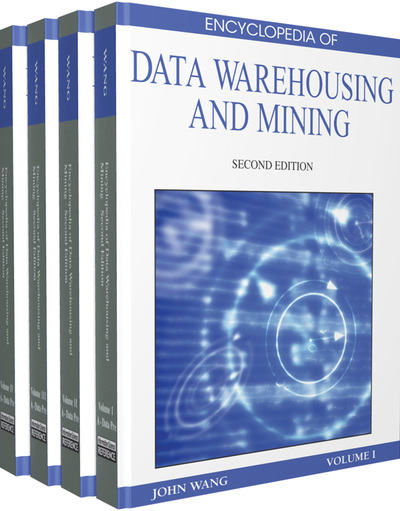# Uncertainty Operators in a Many-Valued Logic

Herman Akdag (LIASD, University Paris 8, France) and Isis Truck (LIASD, University Paris 8, France)
DOI: 10.4018/978-1-60566-010-3.ch305
Available
\$33.75
List Price: \$37.50
10% Discount:-\$3.75
TOTAL SAVINGS: \$3.75

Chapter Preview
Top

## Introduction

This article investigates different tools for knowledge representation and modelling in decision making problems. In this variety of AI systems the experts’ knowledge is often heterogeneous, that is, expressed in many forms: numerical, interval-valued, symbolic, linguistic, etc. Linguistic concepts (adverbs, sentences, sets of words…) are sometimes more efficient in many expertise domains rather than precise, interval-valued or fuzzy numbers. In these cases, the nature of the information is qualitative and the use of such concepts is appropriate and usual. Indeed, in the case of fuzzy logic for example, data are represented through fuzzy functions that allow an infinite number of truth values between 0 and 1. Instead, it can be more appropriate to use a finite number of qualitative symbols because, among other reasons, any arbitrary fuzzification becomes useless; because an approximation will be needed at the end anyway; etc. A deep study has been recently carried out about this subject in (Gottwald, 2007).

In this article we propose a survey of different tools manipulating these symbols as well as human reasoning handles with natural linguistic statements. In order to imitate or automatize expert reasoning, it is necessary to study the representation and handling of discrete and linguistic data (Truck & Akdag, 2005; Truck & Akdag, 2006). One representation is the many-valued logic framework in which this article is situated.

The many-valued logic, which is a generalization of classical boolean logic, introduces truth degrees which are intermediate between true and false and enables the partial truth notion representation. There are several many-valued logic systems (Lukasiewicz’s, Gödel’s, etc.) comprising finite-valued or infinite-valued sets of truth degrees. The system addressed in this article is specified by the use of LM = {τ0,...,τi,...,τM-1} 1 a totally ordered finite set2 of truth-degrees (τi ≤ τj ⇔ i ≤ j) between τ0 (false) and τM-1 (true), given the operators ∨ (max), ∧ (min) and ¬ (negation or symbolic complementation, with ¬τj = τM-j-1) and the following Lukasiewicz implication →L : τiL τj = min(τM-1, τM-1-(i-j))

These degrees can be seen as membership degrees: x partially belongs to a multiset3A with a degree τi if and only if xτi A. The many-valued logic presented here deals with linguistic statements of the following form: x is vαA where x is a variable, vα a scalar adverb (such as “very”, “more or less”, etc.) and A a gradable linguistic predicate (such as “tall”, “hot”, “young”...). The predicate A is satisfiable to a certain degree expressed through the scalar adverb vα. The following interpretation has been proposed (Akdag, De Glas & Pacholczyk, 1992):

x is vαA ⇔ “x is A” is τα – true

## Complete Chapter List

Search this Book:
Reset